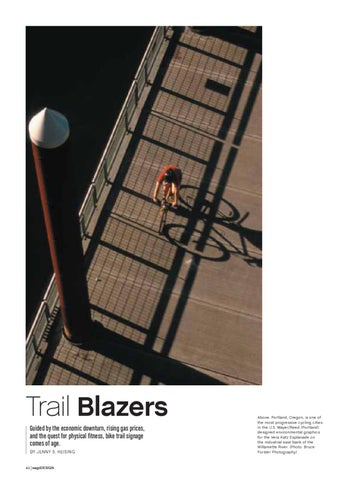# segdDESIGNTrail 8bWp[hi Guided by the economic downturn, rising gas prices, and the quest for physical fitness, bike trail signage comes of age. 8O @;DDO I$H;?I?D= ,( i[]Z:;I?=D 7Xel[0 FehjbWdZ&quot; Eh[]ed&quot; _i ed[ e\ j^[ ceij fhe]h[ii_l[ YoYb_d] Y_j_[i _d j^[ K$I$CWo[h%H[[Z FehjbWdZ Z[i_]d[Z [dl_hedc[djWb ]hWf^_Yi \eh j^[ L[hW AWjp ;ifbWdWZ[ ed j^[ _dZkijh_Wb [Wij XWda e\ j^[ M_bbWc[jj[ H_l[h$ F^eje0 8hkY[ &lt;ehij[h F^eje]hWf^o